# Peak-to-Average-Power-Ratio (PAPR) reduction in WiMAX and OFDM/A systems

## Abstract

A peak to average power ratio (PAPR) reduction method is proposed that exploits the precoding or beamforming mode in WiMAX. The method is applicable to any OFDM/A systems that implements beamforming using dedicated pilots which use the same beamforming antenna weights for both pilots and data. Beamforming performance depends on the relative phase shift between antennas, but is unaffected by a phase shift common to all antennas. PAPR, on the other hand, changes with a common phase shift and this paper exploits that property. An effective optimization technique based on sequential quadratic programming is proposed to compute the common phase shift. The proposed technique has several advantages compared with traditional PAPR reduction techniques in that it does not require any side-information and has no effect on power and bit-error-rate while providing better PAPR reduction performance than most other methods.

## 1. Introduction

Many recent wide-band digital communication systems use a multi-carrier technology known as orthogonal-frequency-division-multiplexing (OFDM), where the band is divided into many narrow-band channels. A key benefit of OFDM is that it can be efficiently implemented using the fast-fourier-transform (FFT), and that the receiver structure becomes simple since each channel or sub-carrier can be treated as narrow-band instead of a more complicated wide-band channel. Orthogonal-frequency-division-multi-access (OFDMA) is a similar technique, but the bands can be occupied by different users.

Although OFDM and OFDMA have many benefits contributing to its popularity, a well-known drawback is that the amplitude of the resulting time domain signal varies with the transmitted symbols in the frequency domain. From OFDM symbol to OFDM symbol, the maximum amplitude can vary dramatically depending on the transmitted symbols. If the maximum amplitude of the time domain signal is large, it may push the amplifier into the non-linear region which creates many problems that reduce performance. For example, it breaks the orthogonality of the sub-carriers which will result in a substantial increase in the error rate. A common practice to avoid this peak-to-average-power-ratio (PAPR) problem is to reduce the operating point of the amplifier with a back-off margin. This back-off margin is selected so that it avoids most of the occurrences of high peaks falling in the non-linear region of the amplifier. Of course, it is desirable to have a minimum back-off margin since operating the amplifier below full power reduces the range of the system, as well as the efficiency of the amplifier.

PAPR reduction is a well-known signal processing topic in multi-carrier transmission and large number of techniques have been proposed in the literature during the past decades. These techniques include amplitude clipping and filtering, coding , tone reservation (TR) [2, 3] and tone injection (TI) , active constellation extension (ACE) [4, 5], and multiple signal representation methods, such as partial transmit sequence (PTS), selected mapping (SLM), and interleaving . The existing approaches differ in terms of requirements and restrictions they impose on the system. Therefore, careful attention must be paid to choose a proper technique for each specific communication system.

WiMAX mobile devices (MS) are commercially available and for the system to work, both mobile devices and basestations need to adhere to the WiMAX standard. Hence, it is not possible to modify the basestation transmission technique if it makes the transmission non-compliant to the standard since existing MS would not be able to decode the transmissions correctly. For example, phase manipulation techniques such as PTS and SLM , which require coded side information to be transmitted would not be compatible or compliant to the standard. One technique of inserting a PAPR reducing sequence is part of the IEEE 802.16e standard. It is activated using the PAPR reduction/sounding zone/safety zone allocation IE. Using this technique reduces the throughput since it requires sending additional PAPR bits. It is also not a part of the WiMAX profile so it is likely not supported by the majority of handsets.

Accordingly, each of the discussed techniques is associated with a cost in terms of bandwidth or/and power. The proposed technique in this paper neither require additional bandwidth nor power while delivering equal or better PAPR reduction gain compared with other existing methods. The proposed algorithm makes use of the antenna beamforming weights and dedicated pilots at the transmitter . It reduces the PAPR by modifying the cluster weights in the WiMAX data structure in a manner similar to the PTS method [7, 8]. The main benefits of the proposed technique are:

• It preserves the transmitted power by adjusting only the phase of the beamforming weights per cluster.

• No extra side information regarding the phase change needs to be transmitted due to the property of dedicated pilots.

• Not sending the phase coefficients allows for arbitrary phase shifts instead of a quantized set such as used for PTS.

• A novel search algorithm based on gradient optimization to find the optimum cluster weights phase shifts.

The following presentation focuses on WiMAX, but the same technique applies to any OFDM/OFDMA system that uses a concept similar to dedicated pilots and does not explicitly announce the multiplied weights to the receiver.

The paper is organized as follows: in Sect.2 the PAPR in an OFDM system is defined, also the data structure in WiMAX profile and potential capabilities of the standard is explained. In Sect.3, the proposed PAPR reduction method is described based on the PTS technique model and the phase optimization problem is formulated. The optimization problem is written as a conventional minimax problem with nonequality constraints in Sect.4 and then a sequential quadratic programming (SQP) technique is proposed to solve the minimax optimization. This approach breaks the complex original problem into several convex quadratic sub-problems with linear constraints. A pseudo code for a tailored SQP approach is given in sect.4-C. Simulation results in Sect.6 confirm the significant PAPR reduction gain applying the SQP algorithm over other techniques, and the complexity evaluation in Sect.5 reveals the advantage of the new optimization method comparing the exhaustive search approach in PTS. Finally, the paper is concluded in Sect.7 with a summary and a brief discussion on further research.

## 2. System Model

Consider an OFDM system, where the data is represented in the frequency domain. The time domain signal s(n), n = 1, 2, ..., N, where N denotes the FFT size is calculated from the frequency domain symbols D(k) using an IFFT as .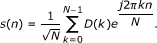(1)

Note that the frequency domain signal D(k) typically belong to QAM constellations. In the case of WiMAX; QPSK, 16QAM and 64QAM constellations are used. The metric that will be used to measure the peaks in the time-domain signal is the PAPR metric defined as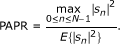(2)

Although not explicitly written in Equation(2), it is well known that oversampling is required to accurately capture the peaks. In this paper, an oversampling of four times is used.

The WiMAX protocol defines several different DL transmission modes, of which the DL-PUSC mode is the most widely used and is on focus here. The minimum unit of scheduling a transmission is a sub-channel, which here spans multiple clusters. One cluster spans 14 sub-carriers over two OFDM symbols containing four pilots and 24 data symbols, which is illustrated in Figure 1. For a 10MHz system, there are a total of 60 clusters. A sub-channel is spread over eight or twelve clusters of which only two or three data sub-carriers from each cluster are used. The sub-channel carries 48 data symbols. For example, logical sub-channel zero uses two data sub-carriers from 12 clusters over two OFDM symbols to reach 48 data symbols.

To extract frequency diversity, the WiMAX protocol specifies that the clusters in a sub-channel are spread out across the band, i.e., a distributed permutation. The WiMAX standard further specifies two main modes of transmitting pilots: common pilots and dedicated pilots. Here, dedicated pilots allow per-cluster beamforming since channel estimation is performed per-cluster, whereas for common pilots channel estimation across the whole band is allowed. The presentation so far has ignored a practical detail of guard bands which are inserted to reduce spectral leakage. In WiMAX, a number of sub-carriers in the beginning and the end of the available bandwidth do not carry any signal, leaving Nusable sub-carriers that carrie data and pilots. Although this number depends on bandwidth and transmission modes, weights that are constant across each cluster are simply applied to only the Nusable sub-carriers.

## 3. Proposed Technique

The proposed technique exploits dedicated pilots for beamforming, which is a common feature in next generation wireless systems. For example, in several 4G systems such as WiMAX  precoding or beamforming weights is not explicitly announced, but instead both pilots and data are beamformed using the same weights. In the WiMAX downlink (DL), beamforming weights are applied in units of clusters (14 sub-carriers), and in the uplink (UL) in units of tiles (four sub-carriers). Beamforming in this context is defined as sending the same message from different antennas, but using different weights per antenna. For a four-antenna BS, the weights can be written as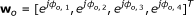where ϕo,1 usually is set to zero for normalization purposes. The beamforming gain for a 4 × 1 channel h becomes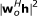. It is clear that we get the same beamforming gain for the vector w = ew o since a phase rotation common to all elements does not change squared product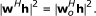However, the common phase rotation has a large impact on the PAPR. Writing the resulting expression for the time-domain signal of the first antenna at tone n using the normalization ϕo,1= 0 yields(3)

where W s (k) denotes the beamforming weight on sub-carrier k, i.e., W s (k) = e(k). Since the channel is estimated using the pilots in each cluster, the beamforming weights need to be constant over each cluster, but can change from cluster to cluster, i.e., W s (k0) = W s (k0 + 1) = ... = W s (k0 + 13), where k0 denotes the first sub-carrier in a particular cluster. In the following, we will focus on the scenario of a single transmission antenna since it simplifies the expressions. However, the method can easily be extended to scenarios with multiple transmit antennas, which is the normal mode of dedicated pilots and beamforming.

For the case of wideband weights, i.e., the beamforming weights are the same across the whole band, the PAPR reduction method is identical and performed only once. For the typical case of narrowband weights, a different beamforming weight per cluster is used so that the PAPR reduction method is applied in a joint fashion over the transmitted signal from all antennas. Furthermore, the technique is readily extendable to single and multi-user MIMO systems using the same concept of dedicated pilots. Although there are now multiple streams, the basestation has to transmit pilots beamformed in the same way as the data. Hence, the same technique as outlined above can be applied. For a basestation sending multiple streams to one or many receivers, the weight optimization now has to be performed jointly over the streams, but otherwise the concept is the same.

The optimization problem of calculating the weights that minimize the PAPR can now be formulated as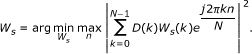(4)

Note that for a 10 MHz WiMAX system, there are 60 clusters so there are 60 phase shifts W s (k) = e(k)where ϕ(k) [0, 2π) and k = 1, 2, ..., 60.

The PAPR reduction technique proposed here is transparent to the receiver and thus does not require any modification to existing receivers and wireless standards. This is clear by writing the received signal z at the handset as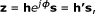(5)

where h' = h ejϕ denotes the effective channel. The BER performance of the effective channel is identical to the original channel. Furthermore, since both pilots and data are transmitted with the same phase shift, the channel estimation performance is also identical. In the proposed technique, the dedicated pilots for channel estimation is used, without interfering with their original job, as an indicator to inform the receiver about the phase rotation at the transmitter. So, the known symbols at allocated subcarriers are phase rotated, as well as data subcarriers. Note that pilot symbols already exists in current design of WiMAX and other similar wireless standards, so we do not reduce the bandwidth for PAPR reduction. The receiver is implicitly informed while the information is hidden at the known pilot symbols. The channel coefficients are estimated for equalization based on received pilots while the PAPR phase rotation is interpreted as the channel effect.

Moreover, the proposed technique does not impact the transmitted power since it is only a phase-modification. In essence, the technique is similar to partial-transmit-sequence (PTS), but without the drawback of requiring side-information which would make it impossible to apply in existing communication standards such as WiMAX. These advantages makes it a very attractive technique to reduce PAPR.

The dedicated pilot feature is designed for beamforming and the standard explicitly states that only the beamformed pilots inside the beamformed clusters can be used for channel estimation and equalization. The weights are different from cluster to cluster. Since only those pilots can be used, there is no other side information that could be used since in the WiMAX case, the phase-change is incorporated into the channel just as any other type of beamforming weights would. Remember that there is no difference between our beamforming weights and normal beamforming weights from a channel estimation perspective. In both cases, there is no need for extra side information. Note that it is possible to design a system different from the WiMAX dedicated pilots setting that could use more side-information, but that is outside the scope of the this paper since it is focusing on WiMAX.

In conclusion, cluster weights can be used to decrease the PAPR of the OFDM symbol. To preserve the average transmitted power, only the phase of the clusters are changed. These phase weights can be multiplied either before IFFT blocks or after it, and the result will be the same due to the linear property of the IFFT operation. However, it is more efficient for the optimization algorithm to apply the phase coefficients after the IFFT block. This is exactly the same approach as the PTS which is explained with a description. However, there are still substantial differences regarding the phase selection, sub-block partitioning, etc.

### A. Partial Transmit Sequence (PTS)

Based on the PTS technique, an input data block of N symbols is partitioned into several disjoint sub-blocks . All elements in each sub-block are weighted by a phase factor associated with it, where these phase factors are selected such that the PAPR of the combined signal is minimized. Figure 2 shows the block diagram of the PTS technique. In the conventional PTS, the input data block D is partitioned into M disjoint sub-blocks D m = [Dm,0, Dm,1, ..., Dm,N-1] T , m = 1, 2, ..., M, such that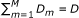, and the sub-blocks are combined to minimize the PAPR in the time domain. The L-times over-sampled time domain signal of D m is obtained by taking an IDFT of length NL on D m concatenated with (L - 1)N zeros, and is denoted by b m = [bm,0, bm,1, ..., bm,LN-1] T , m = 1, 2, ..., M; these are called the partial transmit sequences. Complex phase factors,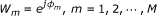are introduced to combine the PTSs which are represented as a vector W = [W1, W2, ..., W M ] T in the block diagram. The time domain signal after combination is given by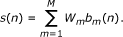(6)

The objective is to find a set of phase factors that minimize the PAPR. In general, the selection of the phase factors is limited to a set with a finite number of elements to reduce the search complexity. The set of possible phase factors is written as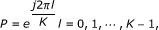where K is the number of allowed phases. The first phase weight is set to 1 without any loss of performance, so a search for choosing the best one is performed over the (M - 1) remaining places. The complexity increases exponentially with the number of sub-blocks M, since KM-1possible phase vectors are searched to find the optimum set of phases. Also, PTS needs M times IDFT operations for each data block, and the number of required side information bits is log2(KM-1) to send to the receiver. The amount of PAPR reduction depends on the number of sub blocks and the number of allowed phase factors .

For each sub-block which is rotated at the transmitter, the applied phase coefficient is sent using a code book to the receiver as an explicit side information which reduce the spectral efficiency. on the other hand, the receiver use the same code book to retrieve the applied phase at the transmitter from side information bits. So the code book needs to be compromised between transmitter and receiver at the system design phase.

PTS performs an exhaustive search among a combination of phase vectors to resolve the optimum weights. For example a permutation of ±1 for two allowed phase factors is performed; in this case, the whole search space for 60 clusters will be 260 alternative vectors, which takes a tremendous amount of computations. Here, we propose a realistic optimization algorithm based on the basic configuration of the PTS sub-blocks.

### B. Formulation of the Phase Optimization Problem

The proposed PAPR reduction method is established based on the PTS model when beamforming weights in WiMAX are the alternatives for phase weights in PTS and the sub-blocks represent the clusters. The matrix B is defined as a NL × M array; it contains the summation of IFFT weights within a cluster. The columns of B are the IFFT output samples of PTS sub-blocks, whose length shows the number of disjoint sub-blocks, and each of them is multiplied with a separate phase weight. A direct calculation to form matrix B costs 60 IFFT blocks of size 1024 which means 60(1024/2) log2(1024) ≈ 3 × 105 complex multiplications. This can be reduced effectively by some interleaving and the Cooley-Tukey FFT algorithm, which is proposed in . The transmitted sequence s is illustrated as a multiplication of matrices B and ϕ in Equation(7).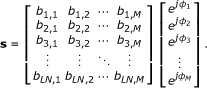(7)

Here, we rewrite the optimization problem to Iind the optimum phase set ϕ as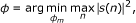(8)

where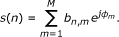(9)

The s(n)s are complex values and ϕ n s are continuous phases between [0, 2π). Substituting b n,m = R n,m + jI n,m and ejϕm = cos ϕ m + j sin ϕ m in Equation(9) and taking the square of |s(n)| results in Equation(10), when R n,m and I n,m stands for {b n,m } and {b n,m } respectively. This is a very important equation, which shows the square of the norm or the power of output sub-carriers that are transmitted; a multi-variable cost function to be minimized when the largest |s(n)| specifies the PAPR of the system. To emphasis on the role of objective function, the |s(n)|2 is replaced with f n (ϕ) as expressed in Equation(10).

Clearly, the multi-variable objective function is continuous and differentiable over [0, 2π), so its gradient can be derived analytically and this is a key property to develop a solution. Knowing the gradient of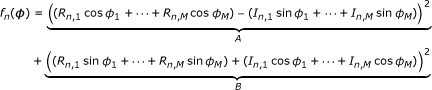(10)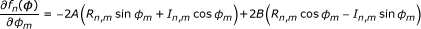(11)

the objective function, the problem can be solved using a wide range of gradient - based optimization methods. The gradient of |s(n)|2 as a function of phase vector ϕ= [ϕ1, ϕ2, , ϕ M ] is defined as the vector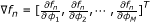. The Jacobian matrix is defined in Equation(12), where M is the number of sub-blocks and LN is the length of the vector s (oversampled OFDM symbol). The n th row of this matrix is the gradient of the f n (ϕ).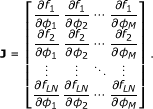(12)

The elements of Jacobian matrix is expressed in Equation (11).

Minimax Approach. The minimax optimization in Equation(8) minimizes the largest value in a set of multi-variable functions. An initial estimate of the solution is made to start with, and the algorithm proceeds by moving towards the minimum; this is generally defined as,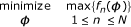(13)

To minimize the PAPR, the objective of the optimization problem is to minimize the greatest value of |s(n)|2 in Equation(9) which is analogous to max{f n (ϕ)} in Equation(13). Here, we reformulate the problem into an equivalent non-linear programming problem in order to solve it using a sequential quadratic programming (SQP) technique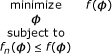(14)

In agreement with this new setting, the objective function f(ϕ) is the maximum of f n (ϕ), or equivalently it is the greatest IFFT sample in the whole OFDM sequence which characterizes the PAPR value. The remaining samples are appended as additional constraints, in the form of f n (ϕ) ≤ f (ϕ). In fact, the f (ϕ) is minimized over ϕ using SQP, and the additional constraints are considered because we do not want other f n s pop out when the maximum value is being minimized. In this way, the whole OFDM sequence is kept smaller than the value that is being minimized during iterations.

## 4. Solving the Optimization Problem

The proposed PAPR reduction technique has unique features of exploiting the dedicated pilots and channel estimation procedure while choosing the best phase coefficients still is a new challenge. In PTS the optimum weights are selected by performing the exhaustive search among the quantized set of phase options, where here there is no restriction on phase coefficients and they can be selected between continuous interval of (0, 2π]. So an efficient optimization algorithm should be used to extract the proper phase choices; the proposed algorithm is a gradient-based method and modified and adapted for the phase optimization problem of the PAPR reduction technique.

SQP is one of the most popular and robust algorithms for non-linear constraint optimization. Here, it is modified and simplified for the phase optimization problem of PAPR reduction, but the basic configuration is as same as general SQP. The algorithm proceeds based on solving a set of subproblems created to minimize a quadratic model of the objective, subject to a linearization of the constraints. The SQP method has been used successfully to many practical problems, see  for an overview. An efficient implementation with good performance in many sample problems is described in .

The Kuhn-Tucker (KT) equations are the necessary conditions for optimality for a constrained optimization problem. If the problem is a convex programming problem, then the KT equations are both necessary and sufficient for a global solution point . The KT equations for the phase optimization problem are stated as the following expression, where λ n s are the Lagrange multipliers of the constraints.(15)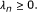(16)

These equations are used to form quasi Newton updating step which is an important step outlined below. The quasi Newton steps are implemented by accumulating second-order information of KT criteria and also checking for optimality during iterations.

The SQP implementation consists of two loops: the phase solution is updated at each fiiteration in major loop with k as the counter, while itself contains an inner QP loop to solve for optimum search direction d k .

Major loop to find ϕ which minimize the f(ϕ):

while k < maximum number of iterations do

ϕk+1= ϕ k + d k ,

QP loop to determine d k for major loop:

while optimal d k found do

dl+1= d l + α d l ,

end while

end while

The step length α is determined within the QP iterations which is distinguished from major iterations by index l as the counter.

The Hessian of the Lagrange function is required to form the quadratic objective function. Fortunately, it is not necessary to calculate this Hessian matrix explicitly since it can be approximated at each major iteration using a quasi Newton updating method, where the Hessian matrix is estimated using the information specified by gradient evaluations. The Broyden Fletcher Goldfarb Shanno (BFGS) is one of the most attractive members of quasi Newton methods and frequently used in non-linear optimization. It approximates the second derivative of the objective function using Equation(17).

Quasi Newton methods are a generalization of the secant method to find the root of the first derivative for multidimensional problems . Convergence of the multi-variable function f(ϕ) can be observed dynamically by evaluating the norm of the gradient |f(ϕ)|. Practically, the first Hessian can be initialized with an identity matrix (H0 = I), so that the first step is equivalent to a gradient descent, while further steps are gradually refined by H k , which is the approximation to the Hessian . The updating formula for the Hessian matrix H in each major iteration is given by,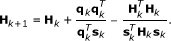(17)

where H is M × M matrix and λ n is the Lagrange multipliers of the objective function f (ϕ).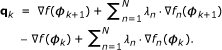(18)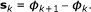(19)

The Lagrange multipliers [according to Equation (16)] is non-zero and positive for active set constraints, and zero for others. The f n (ϕ k ) is the gradient of n th constraints at the k th major iteration. The Hessian is maintained positive definite at the solution point if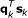is positive at each update. Here, we modifyaq k on an element-by-element basis so that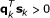as proposed in .

After the above update at each major iteration, a QP problem is solved to find the step length d k , which minimizes the SQP objective function f(ϕ). The complex nonlinear problem in Equation(14) is broken down to several convex optimization sub problems which can be solved with known programming techniques. The quadratic objective function q(d) can be written as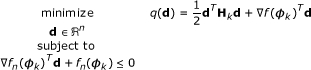(20)

We generally refer to the constraints of the QP sub-problem as G(d) = A d - a, where f n (ϕ k ) T and - f n (ϕ k ) are the n th row and element of the matrix A and vector a respectively.

The quadratic objective function q(d) reflects the local properties of the original objective function and the main reason to use a quadratic function is that such problems are easy to solve yet mimics the nonlinear behavior of the initial problem. The reasonable choice for the objective function is the local quadratic approximation of f(ϕ k ) at the current solution point and the obvious option for the constraints is the linearization of current constraints in original problem around ϕ k to form a convex optimization problem. In the next section we explain the QP algorithm which is solved iteratively by updating the initial solution. The notation in the following section is summarized here for convince.

• d k is a search direction in the major loop while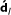is the search direction in the QP loop.

• k is used as an iteration counter in the major loop and l is the counter in the QP loop.

• ϕ k is the minimization variable in the major loop, it is the phase vector in this problem.

• d l is the minimization variable in the QP problem.

• f n (ϕ k ) is the n th constraint of the original minimax problem at a solution point ϕ k .

• G(d l ) = A d l - a is the matrix represents the constraint of the QP sub-problem at a solution point d l and g n (d l ) is the n th constraint.

In a quadratic programming (QP) problem, a multi-variable quadratic function is maximized or minimized, subject to a set of linear constraints on these variables. Basically, the quadratic programming problem can be formulated as: minimizing f(x) = 1/2 xTC x+ cTx with respect to x, with linear constraints Axa ,which shows that every element of the vector Ax is ≤ to the corresponding element of the vector a .

The quadratic program has a global minimizer if there exists some feasible vector x satisfying the constraints, provided that f(x) is bounded in constraints on the feasible region; this is true when the matrix C is positive definite. Naturally, the quadratic objective function f(x) is convex, so as long as the constraints are linear we can conclude the problem has a feasible solution and a unique global minimizer. If C is zero, then the problem becomes a linear programming .

A variety of methods are commonly used for solving a QP problem; the active set strategy has been applied in the phase optimization algorithm. We will see how this method is suitable for problems with a large number of constraints.

In general, the active set strategy includes an objective function to optimize and a set of constraints which is defined as g1(d) ≤ 0, g2(d) ≤ 0, , g n (d) ≤ 0 here. That is a collection of all d, which introduce a feasible region to search for the optimal solution. Given a point d in the feasible region, a constraint g n (d) ≤ 0 called active at d if g n (d) = 0 and inactive at d if g n (d) < 0.b. The active set at d is made up of those constraints g n (d) that are active at the current solution point.

The active set specifies which constraints will particularly control the final result of the optimization, so they are very important in the optimization. For example, in quadratic programming as the solution is not necessarily on one of the edges of the bounding polygon, specification of the active set creates a subset of inequalities to search the solution within . As a result, the complexity of the search is reduced effectively. That is why non-linearly constrained problems can often be solved in fewer iterations than unconstrained problems using SQP, because of the limits on the feasible area.

In the phase optimization problem, the QP subproblem is solved to find the d k vector which is used to form a new ϕ vector in the k th major iteration, ϕk+1= ϕ k + d k . The matrix Q in the general problem is replaced with a positive definite Hessian as discussed earlier, the QP sub-problem is a convex optimization problem which has a unique global minimizer. This has been tested practically in the simulation results, when the d k which minimizes a QP problem with specific setting is always identical, regardless of the initial guess.

The QP subproblem is solved by iterations when at each step the solution is given by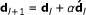. An active set constraints at l th iteration, Á l is used to set a basis for a search direction d l . This constitutes an estimate of the constraint boundaries at the solution point, and it is updated at each QP iteration. When a new constraint joins the active set, the dimension of the search space is reduced as expected.

Theis the notation for the variable in the QP iteration; it is different from d k in the major iteration of the SQP, but it has the same role which shows the direction to move towards the minimum. The search directionin each QP iteration is remaining on any active constraint boundaries while it is calculated to minimize the quadratic objective function.

The possible subspace foris built from a basis Z l , whose columns are orthogonal to the active set Á l , Á l Z l = 0. Therefore, any linear combination of the Z l columns constitutes a search direction, which is assured to remain on the boundaries of the active constraints.

The Z l matrix is formed from the last M - P columns of the QR decomposition of the matrix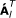Equation(21) and is given by: Z l = Q[:, P + 1: M ]. Here, P is the number of active constraints and M shows the number of design parameters in the optimization problem, which is the number of sub-blocks in the PAPR problem.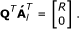(21)

The active constraints must be linearly independent, so the maximum number of possible independent equations is equal to the number of design variables; in other words, P < M. For more details see .

Finally, there exists two possible situations when the search is terminated in QP subproblem and the minimum is found; either the step length is 1 or the optimum d l is sought in the current subspace whose Lagrange multipliers are all positive.

### C. SQP Pseudo Code

Here, a pseudo code is provided for the SQP implementation and we will refer to it in the complexity evaluation section. As discussed in the previous parts, the algorithm consists of two loops.

Step0 Initialization of the variables before starting the SQP algorithm

• An extra element (slack variable) is appended to the variables so ϕ= [ϕ0, ϕ1, ϕ2, , ϕ M ]. The objective function is defined as f(ϕ) = ϕ M and is initialized with zero, other elements can be any random guess.

• The initial Hessian is an identity matrix H0 = I, and the gradient of the objective function is f(ϕ K )T= [0, 0, , 1].

Step1 Enter the major loop and repeat until the defined maximum number of iterations is exceeded.

• Calculate the objective function and constraints according to Equation(10)

• Calculate the Jacobian matrix Equation(11)

• Update the Hessian based on Equation(17) and make sure it is positive definite.

• Call the QP algorithm to find d k

Step2 Initialization of the variables before starting the QP iterations,

• Find a feasible starting point for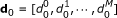and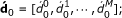Check that the constraints in the initial working setc are not dependent, otherwise find a new initial point d0 which satisfies this initial working set.

Calculate the initial constraints A d0 - a,

if max(constraints) > ε then

The constraints are violated and the new d0 needs to be searched

end if

• Initialize the Q, R and Z and compute initial projected gradient q(d0) and initial search direction d0

Step3 Enter the QP loop and repeat until the minimum is found

• Find the distance in the search direction we can move before violating a constraint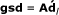(Gradient with respect to the search direction)

ind = find (gsd n > threshold)

if isempty(ind) then

Set the distance to the nearest constraint as zero and put α = 1

else

Find the distance to the nearest constrain as follows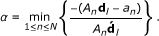(22)

Add the constraint A i d to the active set Á l

Decompose the active set as (21)

Compute the subspace Z l = Q[:, P + 1: M ]

end if

• Update• Calculate the gradient objective at this point Δq(d l )

• Check if the current solution is optimale

if α = 1 || length (Á l ) = M then

Calculate the λ of active set by solving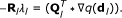(23)

end if

if all λ i > 0 then

return d k

else

Remove the constraints with λ i < 0

end if

• Compute the QP search direction according to the Newton step criteria,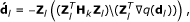(24)

Where theis projected Hessian, see A.

Step4 Update the solution ϕ for the k th iteration; ϕk+1= ϕ k + d k and go back to Step 1

## 5. Complexity Analysis

The SQP algorithm has a quite complicated mathematical concept, and it can be implemented with different modifications. Therefore, the complexity evaluation is not straightforward. The number of QP iterations is not fixedf and is different for each OFDM symbol; here, the average number of QP iterations is considered to evaluate the complexity. For 60 sub-blocks, 1024 sub-carriers and 64 QAM, the average is obtained as 80 iterations for each major SQP iteration.

Another difficulty to compute the required operation is the length of the active set, which alters during iterations starting from 1 to at most M at the end of loop. Consequently, the size of R in the QR decomposition and Z the basis for the search subspace are not fixed during the process so the complexity cannot be assessed directly for each QP iteration and some numerical estimations are necessary.

To evaluate the amount of computation needed for this technique, all steps in the pseudopod are reviewed in detail and an explicit expression is given for each part. First, the complexity of the major loop is assessed in Steps 1 and 4, and then the QP loop is evaluated separately. Finally, the complexity is derived in terms of the number of sub-blocks and major iterations with some approximation and numerical analysis.

Major loop. Steps 1 & 4

1. 1)

Objective function and constraints from Equation(10):

4M × N multiplications and the same amount of addition, N comparisons to find the maximum of constraints

1. 2)

Jacobian matrix from Equation(11):

6M × N multiplications, 4M × N additions

1. 3)

Hessian update Equation(17):

2M × N multiplications, 2M × (N + 1) additions to calculate Equation(19),

3(M + 1) additions and M multiplications for matrices of size M × 1 to compute q k and q k , 2M divisions and M additions are required to update H

1. 4)

The solution ϕ is updated, which requires M additions.

QP loop. Step 3

1. 1)

Gradient with respect to the search direction:

4M × N multiplications and additions to calculate gsd , N comparisons to find the maximum

1. 2)

Distance to the nearest constraint from Equation(22):

2M × N multiplications and additions, N comparisons to find the minimum

1. 3)

Addition of constraint to the active set:

Assume the active set has length L - 1, then the new constraint is inserted and the matrix size becomes M × L. To compute the QR decomposition of this matrix, 2L2(M - L/3) operations are needed .

1. 4)

Update the solution d l which needs M additions.

2. 5)

The gradient objective at the new solution point needs M 2 multiplications and M 2 + 1 additions

3. 6)

The Lagrange multipliers are obtained by solving a linear system of equations, and this impose a complexity in the order of M 3 .

4. 7)

Remove the constraint in case of λ i < 0:

Removing the constraint and recalculation of QR decomposition requires 2L2(M - L/ 3) operations.

1. 8)

Search direction according to Equation(24):

It is a solution to a system of linear equations. The size of Z varies during the iterations, and starts from M × M and reduces to an M × 1 matrix at the end. Accordingly, the complexity in a QP iteration can be stated as 2S2(M + S/ 3) where S is the number of columns in Z at each step.

At first, the computation which is required for the major loop is obtained as 22NM + 9M + N. Next, the amount of computation in the QP loop is divided into fixed and variable partsg; there are (6M + 2)N + 2M2 + M operations which are performed in parts numerated by 1, 2, 4 and 5 in every iterations. Besides there are amount of uncertain operations in other parts which are evaluated separately.

To resolve the search direction in Equation(24) two states is possible: the first M times needs 0.4167M4+ 0.6667M3 + 0.25M2 operations, which is derived by numerical analysis and polynomial fitting, and for further iterations each needs 2M operations. Therefore the required number of flops can be approximated as 0.4M3+0.7M2+0.2M for each iteration. In the QR decomposition part, which is certainly done in every iterations, the procedure is the same. It means that for the first M iteration, 0.25M4 - 0.3333M3 + 0.0833M2 operations and for the extra ones 4/3M3 flops are done. So the amount of major computation is approximated to be 0.25M3 for each QP iteration by dividing the total operations over M.

With an acceptable approximation, we claim that the Lagrange multipliers calculation can be neglected in comparison with other dominant parts of computations, because it mostly appears after M +1 iterations; this occurs when the active constraints are full (M constraints are added to the active set), or sometimes when the exact step to the minimum is found. To sum up, the total number of operations needed for each QP iteration is roughly expressed as 0.65M3 + 2.7M2 + 6NM + 2N, and the total complexity is shown in Table 1, where k and l are the number of major and QP iterations respectively.

There are other optimization methods that can be used to find the best phase weights. PSO is one of the proposed methods for PTS phase search algorithm and many modifications have been introduced to simplify the technique . But the numerical optimization techniques like PSO are only applicable for PTS with limited number of sub-blocks and subcarriers (at most 256 subcarriers and 16 sub-blocks) so that the algorithm converges fast enough to the optimal solution. But here there are 60 sub-blocks and when the allowed phase set is just ±1, the initial generated solutions span 260 possible options. To reduce the convergence time of the optimization technique, the number of randomly generated solutions needs to be a reasonable proportion of all possible solutions, while the complexity is increased linearly with the number of particles in the initial swarm population. The continuous version of PSO is implemented and simulation result is shown in Figure 7 when the number of computations is almost equal to the generated SQP curve.

The complexity of PSO is expressed as the number of required flops in Table 1 where k is the number of iterations and n is the number of initial solutions or the swarm population. For more details on the complexity of PSO, see .

The complexity of SQP is graphically illustrated, showing the number of operations in the SQP algorithm for two OFDM symbols in time with 1024 sub-carriers. Figure 3 indicates the trend when the number of iterations increases. Predictably, when more sub-blocks are chosen to be phase rotated, then the complexity is raised with sharper slope versus the number of iterations, because M3 is the coefficient which dominantly defines the slope of l. Figure 4 shows how the complexity grows almost linearly with the number of sub-blocks for less number of iterations, while it tends to a cubic curve for larger number of iterations.

The exhaustive search whose complexity is shown in the first row of Table 1 is used in conventional optimal PTS and has a significantly higher cost compared to the proposed algorithm. Moreover, the performance is not as good as SQP, since the phase coefficients are optimized among a quantized phase set. The whole calculation in Equation(7) has to be repeated for every combination of phase vectors, and this requires KM × MN times additions and multiplications, where K is the number of allowed phases and M is the number of sub-blocks. Additionally, KM × (N + 1) comparisons are needed to find the largest sample among each produced transmit sequence, and also between all PAPRs to choose the minimum.

To have a better perception of the PTS complexity in this context, assume the allowed phase set is ±1, so K = 2 and no phase rotation required. Also, the number of sub-blocks is M = 60 and the same setting preserved as the SQP; then approximately, 1023 additions and 1021 comparisons have to be performed to find the optimum phase which is clearly impractical. In contrast, the SQP requires 108 flops for 60 sub-blocks which is roughly equivalent to the PTS exhaustive search with only 12 sub-blocks and two phase options. According to the recent developments in DSP technology and time schedule in WiMAX and LTE standard, this amount of computation is affordable.

There are many methods in the literature which is dedicated to develop sub-optimal PTS schemes to reduce the complexity of exhaustive search in conventional PTS technique, in cost of performance degradation. In this paper, we introduced a systematic optimization technique to achieve the optimal solution of phase rotation approach for PAPR reduction, which has not been studied before. Also, the proposed technique does not require any common costs in terms of increasing BER in the receiver or transmit power, so the costly part is just the optimization procedure. While in every other PTS techniques, the side information is sent to the receiver which cause the spectral efficiency reduction, increasing the transmit power or even BER degradation in case of transmission error.

There are not many options for PAPR reduction techniques without side information and it is not fair to compare SQP technique with other PTS phase optimization approaches which require explicit information to be sent to the receiver.

## 6. Simulation Results

The proposed PAPR reduction technique for an OFDMA system with 1024 sub-carriers and 64 QAM modulation is simulated for a WiMAX data structure as explained in Figure 1. The cumulative distribution function (CDF) of the PAPR is one of the most frequently used performance measures for PAPR reduction techniques. The complementary CDF (CCDF) is used here to evaluate different methods, which denotes the probability that the PAPR of a data block exceeds a given threshold and is expressed as CCDF = 1 - CDF.

To have a better perception of the PAPR cost function, a 3-D plot is provided in Figure 5, which illustrates the variation of PAPR, or equivalently the maximum amplitude of one OFDM symbol partitioned into two disjoint sub-blocks, versus two phase coefficients. Predictably, two sub-blocks cannot do much for the PAPR reduction purpose and this is just to give a visual impression of the cost function to be minimized in the SQP optimization algorithm.

As can be seen, there are many local minima which have slightly different levels; that is one of the promising properties of this optimization problem because reaching a local minimum satisfies the PAPR reduction aim even though the global minimum is not found. As a result, the performance of the proposed algorithm is relatively insensitive to the initialization of the optimization.

The time domain signal of two 1024-OFDM symbol is shown in Figure 6, before and after the signal processing algorithm. It is clear that the proposed method reduces the magnitude variations dramatically and that the back-off margin can be much smaller.

### A. Performance of Different Algorithms

The performance of four different optimization techniques is illustrated in Figure 7 by CCDF curves. Once the Jacobian of the cost function is defined, the optimization problem can be treated with different optimization methods. The SQP is the best solution for the problem in terms of PAPR reduction performance, but the least square error (LSE) approach can also be used to reduce the peak amplitude of the signal with much less complexity. However, the performance is not as good as the SQP algorithm but still comparable with existing PAPR reduction techniques .

The LSE algorithm minimizes the objective function f(x) = (f1(x))2+(f2(x))2+ +(f N (x))2, which is the sum of the OFDM sub-carriers amplitudesh. The components are forced to be equal to minimize the sum, so the large samples are pushed to a specific level, whereas the smaller ones become larger. One of the examined optimization methods to search the phase coefficients in PTS is particle swarm optimization (PSO) . The achieved gain for PSO is slightly better than LSE, but it is expensive to implement especially when the number of sub-blocks is large. The simulation results shows for the same amount of computation the PSO is 2dB worse than SQP, when the initial particle number is n = 100 and k = 50 iterations .

If the search for the global minimum can be performed in each OFDM symbol, then the CCDF curve improves to some degree. In our test, each OFDM symbol has been processed 100 times with different initial guesses and the one with the smallest PAPR is selected. The result in Figure 7 (advanced SQP) shows an overall improvement of about 0.5 dB. In this case, the PAPR of the system can almost be considered as a deterministic value since the CCDF curve is almost vertical.

### B. Evaluation of Effective Parameters in SQP Performance

Figure 8 shows the performance of the SQP algorithm at the point Pr{PAPR > PAPR0} = 10-4 for 10,000 random OFDM symbol with 64 QAM modulation versus different number of major iterations. The vertical axis represents the PAPR reduction gain in dB, which is the difference between the original CCDF curve and the processed signal curve at the probability as indicated in Figure 7. As noticed here, most of the job is done in the first iteration and after more than ten iterations the progress tends to be slower.

Figure 9 shows the PAPR reduction degradation, when the number of sub-blocks are reduced. As explained earlier, each cluster can be phase rotated and this will be reversed at the receiver in the channel equalization process. To bring down the complexity, the same phase coefficients are assigned to several adjacent clusters to simplify the optimization algorithm. In fact, 30 sub-blocks means two clusters within one sub-block and each sub-block is weighted with specific phase coefficient. In practice, there cannot be 120 phase coefficients or sub-blocks, because it means that one cluster has two phase weights and this is not possible to compensate at the receiver according to the WiMAX standard. But in Figure 9, a 120 sub-blocks configuration is simulated to show the trend of PAPR reduction gain versus the number of sub-blocks.

Finally, the PAPR reduction performance in terms of CCDF curve is not changed with different initial guesses, because the maximum of all 10, 000 simulated OFDM symbols defines the CCDF curve in low probability of Pr{PAPR > PAPR0}, and this does not depend on the initial solution. But in each OFDM symbol the minimum can be found by examination of various starting points and the performance can be improved as Figure 7 illustrates in advanced-SQP curve.

## 7. Concluding Remarks

We introduced a precoding PAPR reduction technique that is applicable to OFDM/A communication systems using dedicated pilots. We developed the technique for a WiMAX system but it is applicable to OFDM/A systems in general where dedicated pilots and data both are beamformed. Beamforming performance depends on the relative phase shift between antennas but is unaffected by a phase shift common to all antennas. PAPR, on the other hand, changes with a common phase shift, and the PAPR reduction technique proposed in this paper was based on this property. Each cluster within the WiMAX data structure are weighted with proper phase coefficients, which are optimized to minimize the PAPR of the time domain transmitted signal.

The proposed technique comes with interesting unique features, making it a very appealing method especially for standard constrained applications. No side information is sent to the receiver so the throughput is not affected and transmitted power and bit error rate does not increase which otherwise are common drawbacks in many PAPR reduction techniques. Moreover, an optimization technique for finding the best weights was proposed. The PAPR reduction problem was formulated as a minimax problem that was solved by deriving the gradient analytically and modifying the SQP algorithm to solve the optimization.

The SQP algorithm works effectively with a large PAPR reduction gain. At the cost of a smaller PAPR reduction gain, it is possible to reduce the computational complexity of the technique by using other gradient-based optimization techniques. Even lower complexity can be achieved using a least squares-based formulation, but simulation results indicated a substantial performance loss compared with the SQP approach. The SQP itself can be implemented in different ways to simplify the algorithm and several steps can be done in parallel for a more practical hardware implementation.

## Appendix A

### Calculation of the search directionThe procedure of deriving search direction of the QP is explained in  and included here for convenience. Once Z l is derived, a new search directionis updated that minimizes the QP objective function q(d), which is a linear combination of the columns of Z l and located in the null space of the active constraints. Thus, the quadratic objective function can be reformulated as a function of some vector b by substituting for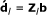, in general QP problem.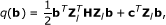(25)

Differentiating with respect to b yields,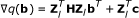where q(b) is referred to as the projected gradient of the quadratic function, because it is the gradient projected to the subspace defined by Z l . The minimum of the function q(b) in the subspace defined by Z l occurs when q(b) = 0, which is the solution of the system of linear equations.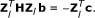(26)

Solving Equation(26) for b at each QP iteration gives the, then the step is taken as. Since the objective is a quadratic function, there are only two choices of step length α; it is either 1 along search directionor < 1. If the step length 1 can be taken without violation of the constraints, then this is the exact step to the minimum of the quadratic function. Otherwise, the distance to the nearest constraint should be found and the solution is moved along it as in Equation(22).

## Endnotes

aThe general aim of this modification is to distort the elements of q k , which contribute to a positive definite update, as little as possible. Therefore, in the initial phase of the modification, the most negative element ofis repeatedly halved. This procedure is continued untilis greater than or equal to a small negative tolerance. If, after this procedure,is still not positive, modify q k by adding a vector v multiplied by a constant scalar w, and increase w systematically untilbecomes positive see . bEquality constraints are always active but there is no equality constraints in this phase optimization problem. cWhen it is not the first major iteration, the active set is not empty. dWhere i is the index of minimum in (22) which indicates the active constraint to be added. eThe term "length" indicates the number of rows in A l or equivalently the number of active constraints. fThe QP is a convex optimization problem, so the iterations proceed till the optimum is found, but a modification of the algorithm can be used when the number of iterations are fixed. gThe fixed operations belong to those matrices whose sizes do not change during the iterations while there are other matrices like Z that has variable size and hence different complexity during iterations. hThis is the simplest scenario, but other modifications can be made to develop a more elaborate version of LSE.

## References

1. 1.

Patterson K: Generalized reed-muller codes and power control in OFDM modulation. IEEE Trans Inf Theory 1997, 46: 104-120.

2. 2.

Tellado J: Multicarrier Modulation with Low Peak to Average Power Applications to xDSL and Broadband Wireless. Kluwer Academic, Norwell, MA; 2000.

3. 3.

Behravan A: Evaluation and Compensation of Nonlinear Distortion in Multicarrier Communication Systems. PhD thesis. Chalmers University of Technology, Department of Signals and Systems, Communication System Group, Gothenburg, Sweden; 2006.

4. 4.

Ciochina C, Buda F, Sari H: An analysis of OFDM peak power reduction techniques for WiMAX systems. Proceedings of IEEE International Conference on Communications 2006, 46: 104-120.

5. 5.

Krongold BS, Jones DL: PAR reduction in OFDM via active constellation extension. IEEE Trans Broadcast 2003, 3: 258-268.

6. 6.

Han SH, Lee JH: An overview of peak-to-average power ratio reduction techniques for multicarrier transmission. IEEE Wirel Commun Mag 2005, 12: 56-65. 10.1109/MWC.2005.1421929

7. 7.

Tellambura C: Phase optimization criterion for reducing peak-to-average power ratio in OFDM. IET Electron Lett 1998, 34: 169-170. 10.1049/el:19980163

8. 8.

Cimini LJ Jr, Sollenberger NR: Peak-to-average-power ratio reduction of an OFDM signal using partial transmit sequences. IEEE Commun Lett 2000, 4: 86-88. 10.1109/4234.831033

9. 9.

Mller SH, Huber JB: A novel peak power reduction scheme for OFDM. Proceedings of IEEE PIMRC 1997, 3: 1090-1094.

10. 10.

Andrews JG, Ghosh A, Muhamed R: Fundamentals of WiMAX: Understanding Broadband Wireless Networking. Prentice Hall; 2007.

11. 11.

Kang S, Kim J, Joo E: A novel sub-block partition scheme for partial transmit sequence OFDM. IEEE Trans Commun 1999, 45: 333-338.

12. 12.

Fletcher R: Practical Methods of Optimization. 2nd edition. Wiley-Interscience; 2000.

13. 13.

Gill P, Murray W, Wright MH: Practical Optimization. Academic Press; 1981.

14. 14.

Powell MJD: Variable Metric Methods for Constrained Optimization. Springer Verlag; 1983.

15. 15.

Schittkowski K: NLQPL: a FORTRAN-subroutine solving constrained nonlinear programming problems. Ann Oper Res 1985, 5: 485-500.

16. 16.

Kuhn HW, Tucker AW: Nonlinear programming. Proceedings of Second Berkeley Symposium on Mathematical Statistics and Probability 1951, 481-492.

17. 17.

Yi Z: Ab-initio Study of Semi-conductor and Metallic Systems: From Density Functional Theory to Many Body Perturbation Theory. PhD thesis. University of Osnabruck, Department of Physics, Osnabruck, Germany; 2009.

18. 18.

Amjady N, Keynia F: Application of a new hybrid neuro-evolutionary system for day-ahead price forecasting of electricity markets. Appl Soft Comput 2010, 10: 784-792. 10.1016/j.asoc.2009.09.008

19. 19.

Matlab optimization toolbox user guide, constrained optimization Volume ch 6. The MathWorks, Inc; 1984:227-235.

20. 20.

Murty KG: Linear Complementarity, Linear and Nonlinear Programming, Sigma Series in Applied Mathematics. Volume 3. Heldermann Verlag, Berlin; 1988.

21. 21.

Gill P, Murray W, Wright M: Numerical Linear Algebra and Optimization. Volume 1. Addison-Wesley; 1991.

22. 22.

Nocedal J, Wright SJ: Numerical Optimization. Operations Research and Financial Engineering. 2nd edition. Springer Verlag; 2006.

23. 23.

Qu YJ, Hu BG: RBF networks for nonlinear models subject to linear constraints. IEEE International Conference on Granular Computing 2009, 482-487.

24. 24.

Golub GH, Loan CV: Matrix Computations. 3rd edition. Johns Hopkins University Press, Baltimore, MD; 1996.

25. 25.

Wang Y, Chen W, Tellambura C: A PAPR reduction method based on artificial bee colony algorithm for OFDM signals. IEEE Trans Wirel Commun 2010, 9: 2994-2999.

26. 26.

Khademi S: OFDM peak-to-average-power-ratio reduction in WiMAX systems. In Master's thesis. Chalmers University of Technology, Department of Signals and Systems, Communication System Group, Gothenburg, Sweden; 2011.

27. 27.

Kennedy J, Eberhart R: Particle swarm optimization. Proceedings of IEEE International Conference on Neural Networks 1995, 46: 1942-1945.

## Author information

Authors

### Competing Interests

The authors declare that they have no competing interests.

S K was born on September 22, 1981 in Kermanshah, Iran. She received the B.S. degree in Electrical Engineering with Communication minor, in 2005 from State University of Tabriz in Iran with dissertation in the field of satellite communication titled as frequency reuse in dual polarized satellite systems. She got her M.S. degree in Communication Engineering from Chalmers University of Technology in Gothenburg, Sweden in 2010. She is a PhD student at Delft University of Technology now and working on signal processing applications for wireless communication, her master thesis was related to PAPR reduction techniques for WiMAX systems.

T S (S'98,M'01) was born in Trollhättan, Sweden, in 1972. He received the M.S. degree in electrical engineering in 1996 and the Ph.D. degree in signal processing in 2001 from Chalmers University of Technology, Gothenburg, Sweden. During 2001-2005 he conducted research on adaptive antennas in wireless communications, channel modeling and probing of systems employing transmit and receive antenna arrays at Brigham Young University (BYU) and University of California, San Diego (UCSD). Since 2005, he is with ArrayComm, San Jose, CA developing adaptive antenna algorithms for emerging broadband technologies such as WiMAX and 3GPP LTE.

M V received the PhD degree in Automatic Control from Linköping University, Sweden in 1989. He has held academic positions at Linköping University and visiting Scholarships at Stanford University and Brigham Young University, USA. Since 1993, Dr. Viberg is a professor of Signal Processing at Chalmers University of Technology, Sweden. During 1999-2004 he served as Department Chair. Since May 2011, he holds a position as Vice President of Chalmers university of Technology. Dr. Viberg's research interests are in Statistical Signal Processing and its various applications, including Antenna Array Signal Processing, System Identification, Wireless Communications, Radar Systems and Automotive Signal Processing. Dr. Viberg has served in various capacities in the IEEE Signal Processing Society, including chair of the Technical Committee (TC) on Signal Processing Theory and Methods (2001-2003), vice-chair (2009-2010) and chair (from 2011) of the TC on Sensor Array and Multichannel, Associate Editor of the Transactions on Signal Processing (2004-2005), member of the Awards Board (2005-2007), and member at large of the Board of Governors (2010-). Dr. Viberg has received 2 Paper Awards from the IEEE Signal Processing Society (1993 and 1999 respectively), and the Excellent Research Award from the Swedish Research Council (VR) in 2002. Dr Viberg is a Fellow of the IEEE since 2003, and his research group received the 2007 EURASIP European Group Technical Achievement Award. In 2008, Dr. Viberg was elected into the Royal Swedish Academy of Sciences (KVA).

T E was born on April 7, 1964 in Skovde, Sweden. He received the M.Sc. degree in Electrical Engineering in 1990, and the Ph.D. degree in Information Theory in 1996, both from Chalmers University of Technology, Gothenburg, Sweden. He was at AT&T Labs - Research from 1997 to 1998, and in 1998 and 1999 he was working on a joint research project with the Royal Institute of Technology and Ericsson Radio Systems AB. In 2003 and 2004, he was a guest professor at Yonsei University in Seoul, South Korea. Currently, he is an Associate Professor (docent) at the department of Signals and Systems, Chalmers University of Technology. His research interests include communication systems, source coding and information theory. Specific interests include multiple description quantization, speaker recognition, channel quality feedback, and system modeling of non-ideal hardware components.

## Authors’ original submitted files for images

Below are the links to the authors’ original submitted files for images.

## Rights and permissions

Reprints and Permissions

Khademi, S., Svantesson, T., Viberg, M. et al. Peak-to-Average-Power-Ratio (PAPR) reduction in WiMAX and OFDM/A systems. EURASIP J. Adv. Signal Process. 2011, 38 (2011). https://doi.org/10.1186/1687-6180-2011-38

• Accepted:

• Published:

### Keywords

• WiMAX
• OFDM
• PTS
• PAPR reduction
• phase optimization